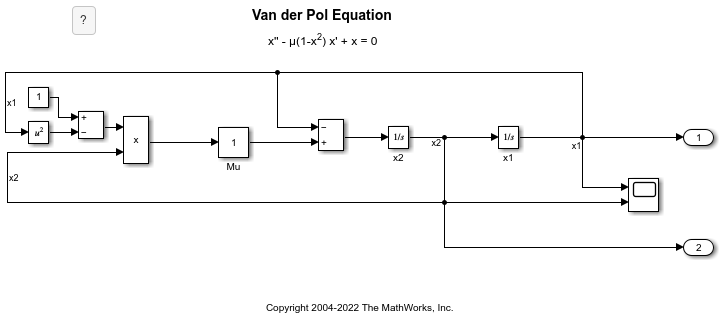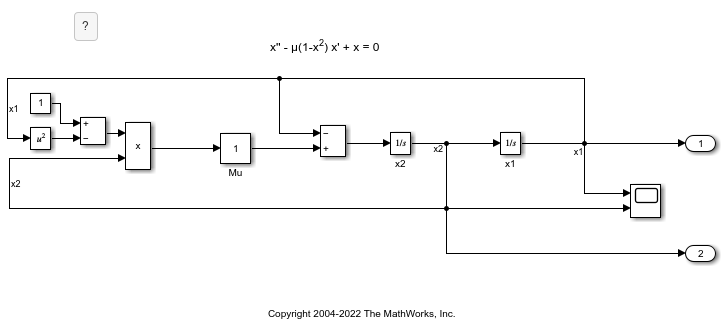# delete

Delete annotation

## Syntax

``delete(a)``

## Description

example

````delete(a)` deletes the specified annotation.```

## Examples

collapse all

This example shows how to delete an annotation in the `vdp` model programmatically.

Open the example. Then, open the `vdp` model.

`open_system('vdp')`To get the handles for the annotations in the model, use the `find_system` function.

`h = find_system(gcs,'FindAll','on','Type','annotation');`

To identify the annotations, query the text inside the annotations.

`get_param(h,'PlainText')`
```ans = 3x1 cell {'Copyright 2004-2022 The MathWorks, Inc.'} {'Van der Pol Equation' } {'x'' - µ(1-x^2) x' + x = 0' } ```

To delete the title of the model, `'Van der Pol Equation'`, get the `Simulink.Annotation` object that corresponds to the second handle.

`an = get_param(h(2),'Object');`

Delete the annotation from the model.

`delete(an)`Get the handles for the annotations in the model. Then, query the text inside the annotations.

```h1 = find_system(gcs,'FindAll','on','Type','annotation'); get_param(h1,'PlainText')```
```ans = 2x1 cell {'Copyright 2004-2022 The MathWorks, Inc.'} {'x'' - µ(1-x^2) x' + x = 0' } ```

The model no longer has an annotation with `'Van der Pol Equation'` as text.

## Input Arguments

collapse all

Annotation, specified as a `Simulink.Annotation` object.

## Version History

Introduced before R2006a